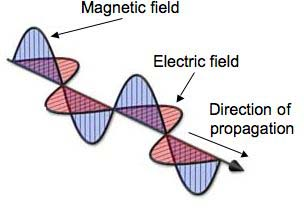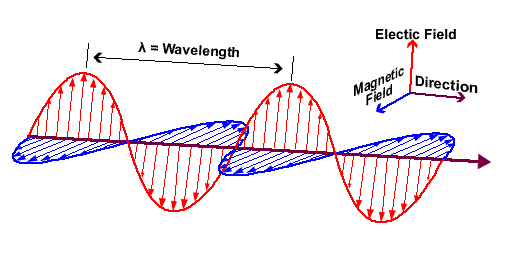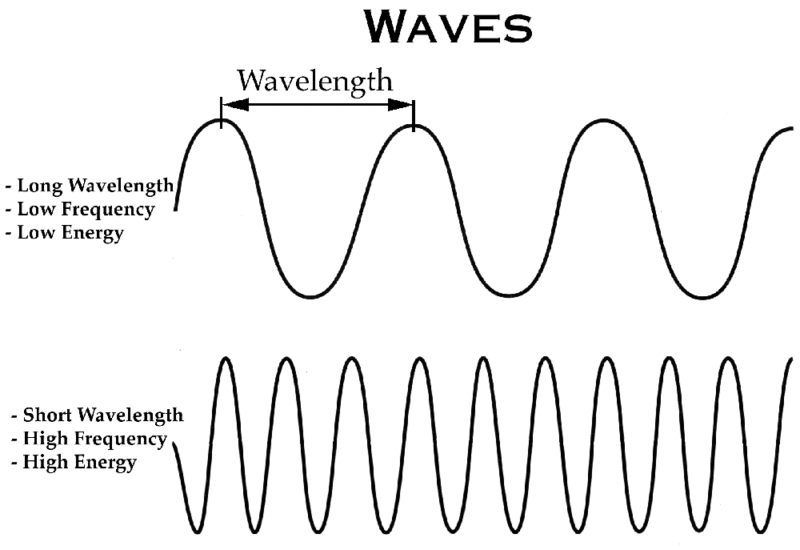# Transmission Basics: Beginners Guide To EM Waves

11359
Advertisement

Among the different types of electromagnetic or EM waves, radio signals are used very widely in our lives. Cue in this widespread usage, they have a major effect on RF antennas themselves and RF antenna design.EM waves field directions

RF, visible, ultra-violet and infrared all comprise electromagnetic waves differing from each other in wavelength and frequency. These are made of both electric and magnetic components, which are inseparable. Forming a 3D structure, the individual fields are at right angles to one another and to the direction of motion of the wave at the same time.

In antennas, electric fields generally result from voltage changes in RF radiated signal whereas the magnetic changes cue into the current flow. The electric field lines often run along the same axis as the RF antenna, spreading out as they move away. It is measured in terms of the change in potential, over a given distance, e.g. volts per metre, and is hence called field strength. Similarly on reception of a signal the magnetic changes would cause a current flow, resulting in an electric field, which in turn causes voltage changes on the antenna.

# Wave PropertiesEM wavelength

Among the major properties of a wave, the first is its wavelength, measured as the distance between two similarly identical points. To resolve any confusion the previous line might have made, we can measure the wavelength from one peak to the peak, or one trough to the next. A peak is the highest or the positive maximum value, whereas a trough is the lowest or the negative highest value of the signal. Since these are easy to identify in a wave, the wavelength can be very easily calculated.

Advertisement

Frequency is another important property of a wave. It can be calculated by the number of waves in a certain specified time period. Generally, this time period is taken as a second. The unit of frequency, Hertz, is named after the German scientist who discovered radio waves. Coming back to RF waves, the prefixes to frequencies Kilo, Mega, and Giga are often seen, since these are very high.

RF travel at the speed of light. For calculation purposes, it is considered at 300 million metres per second, whereas the exact value would be a little less.

## Frequency-Wavelength CorrelationRelating wavelength frequency and energy

Frequency is often used as a measure of the signal. Frequency and wavelength are relate to each other by the below relation:

λ = c / f

where

λ is the wavelength, in metres
f is frequency, in Hertz
c is the speed of light, in metre / second

## Field measurements

Similar to a transformer, there is also an inductive field close to the RF antenna. This can often cause distortions in the original measurements in the nearby signals since this is not the intended signal. This often becomes a hassle with multiple antenna transmitting at close proximity. This causes interference at the receiving end of the antenna as well. Additionally, the wiring for electricity and other transfer might also cause signal distortions. The saving grace here would be the fact that, it starts to fade away after a couple of meters.

Advertisement